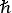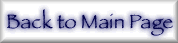## 4. Basic Transform Equations

Appropriate formulae based on (eq 7) should give a small and smooth integral over the range of the integrating variable, with the dominant term given by analytic form. The situation may be illustrated by considering the above-edge case, given by Eq. 27 of Ref. :(eq 8)

with α the fine structure constant and energies and σ in atomic units (au). Gauss-Legendre integration points are fixed by the order of the integration, but corresponding energies may be adjusted to optimize the precision for a particular photon energy by scaling (contracting or expanding) the integrating variable, following (but in contrast to) Eq. 29 of Ref.  [for Eq. (8)]:(eq 9)

Note that ε+>0 and -ε1>0 and the integration limits are transformed from (0,∞) to (1,0), while in the first case σ(ε+ - ε1) becomes σ(- ε1/x). By symmetry, this yields a well-defined integral which properly ranges from 0 to 1.

Following the same formalism as in [17-20], improved if not fully optimized relations for f+ may then be given as(eq 10)(eq 11)(eq 12)(eq 13)(eq 14)

forω > |ε1| (above the edge). At the edge, use(ω + δω) to avoid the computational singularity. Below the edge (|ε1| >ω),(eq 15)(eq 16)(eq 17)(eq 18)

where, again, σ(|ε1|) is given by σ(|ε1|(1+δ)) to arbitrary precision. The second terms of (eq 10) and (eq 15) are always negative, while the first term in (eq 10) is positive and the first term in (eq 15) is negative. Use of (these) appropriate functions can lead to problems where integration points would lie well beyond the database (11 data points per orbital). In such cases, the above-edge or below-edge behaviour should be retained, but the choice of form may be modified appropriately to match the data and to optimize precision. The effects of this formalism and these specific modifications, compared to the earlier results, may be seen generally near edges, at energies below 2 keV or above 70 keV, but also at intermediate energies.